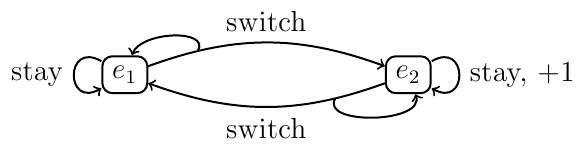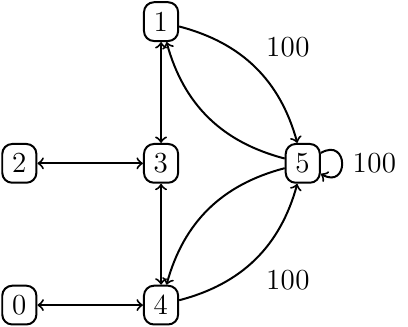Warning
This page is located in archive.

# Tutorial 9 - reinforcement learning III.

## Problem 1 - Passive reinforcement learning

Consider the following MDP. Assume that reward is in the form $r(e,y)$, i.e., $r: E \times Y \mapsto \mathbb{R}$. Set $\gamma = \frac{1}{2}$.Suppose that you have seen the following sequence of states, actions, and rewards: $$e_1, \mathrm{switch}, e_2, \mathrm{stay}, +1, e_2, \mathrm{stay}, +1, e_2, \mathrm{switch}, e_1, \mathrm{stay}, e_1, \mathrm{switch}, e_1, \mathrm{switch}, e_1, \mathrm{stay}, e_1, \mathrm{switch}, e_2, \mathrm{stay}, +1, {\color{lightgray} e_{\color{lightgray} 2}}$$

1. What is $\hat{U}^{\pi}(e_i)$ calculated by the Direct Utility Estimation algorithm?
2. What is transition model $\hat{\mathcal{E}}$ estimated by the Adaptive Dynamic Programming algorithm?
3. What are state values estimated by a Temporal Difference learning agent after two steps? Assume that $\alpha=0.1$ and all values are initialized to zero.

## Problem 2 - Greedy policy and value function

Decide whether the following statement is true or false: If a policy $\pi$ is greedy with respect to its own value function $U^{\pi}$, then this policy is an optimal policy.

## Problem 3 - GLIE and convergence

Do the following exploration/exploitation schemes fulfill the 'infinite exploration' and 'greedy in limit' conditions? Which lead to convergence of $Q$-values in $Q$-learning and which lead to convergence of $Q$-values in SARSA. Does anything change if we are interested in the convergence of policy? $N_{e,y}$ denotes the number of times when action $y$ was taken in state $e$. $N_e$ is defined similarly.

1. a random policy
2. $$\pi(e) = \begin{cases} y, & \mbox{if } N_{e,y} \leq 100, \\ \mathop{\mathrm{arg\, max}}_y Q(e,y), & \mbox{otherwise}.\end{cases}$$
3. $\varepsilon$-greedy policy with $\varepsilon = \frac{1}{N_e^2}$
4. $\varepsilon$-greedy policy with $\varepsilon = \frac{1,000}{999+N_e}$
5. $\varepsilon$-greedy policy with $\varepsilon = \frac{1}{\sqrt{N_e}}$

Consider the following MDP with $\gamma=0.8$, $r(5) = 100$, $r(\cdot) = 0$.The initial matrix of $Q$-values is $$Q(e,y) = \begin{bmatrix} - & - & - & - & 0 & - \\ - & - & - & 0 & - & 0 \\ - & - & - & 0 & - & - \\ - & 0 & 0 & - & 0 & - \\ 0 & - & - & 0 & - & 0 \\ - & 0 & - & - & 0 & 0 \end{bmatrix}.$$ Consider path $1-5-1-3$ and constant learning rate $\alpha = 0.1$. Show changes in $Q$ values after the agent-environment interaction.

Problems 1,2, and 4 were adapted from Richard Sutton's 609 course. You may find the related materials on http://www.incompleteideas.net/book/the-book-2nd.html.

courses/smu/tutorials/tutorial9.txt · Last modified: 2019/04/15 12:22 by rysavpe1